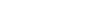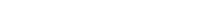When do we use the Present Simple?, When do we use the Present Continuous?, When do we use the Past Simple?, Make a positive sentence using the Present Simple., Make a negative sentence using the Present Continuous., Make a sentence using ALWAYS + the Present Simple. , Make a sentence using ALWAYS + the Past Simple. , Name a verb that add -es to form the Present Simple for the 3rd person singular., Name 1 verb that adds -ies to form the Present Simple for the 3rd person singular., Name 1 verb that add -s to form the Present Simple for the 3rd person singular. , Make a negative sentence using the Present Continuous. , Make a negative sentence using the Present Simple., Make a negative sentence using the Past Simple., Make a question using the Present Simple., Make a question using the Present Continuous., Make a question using the Past Simple., Make a positive sentence using the future meaning of the Present Continuous., Make a negatiive sentence using the future meaning of the Present Continuous., Make a question using the future meaning of the Present Continuous., Make a positive sentence using the Past Simple..

# Tense Review - Present Simple, Present Continuous and Past Simple

Random wheel is an open-ended template. It does not generate scores for a leaderboard.

### Options### Switch template

#### Interactives